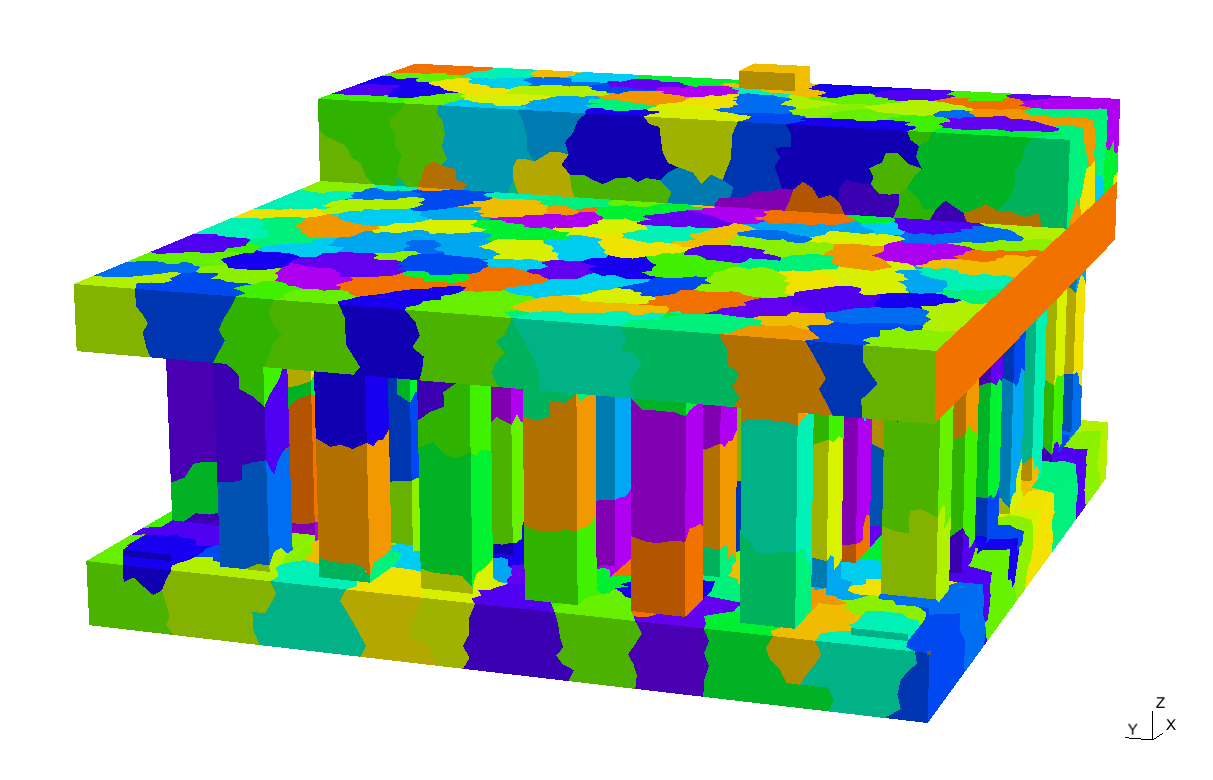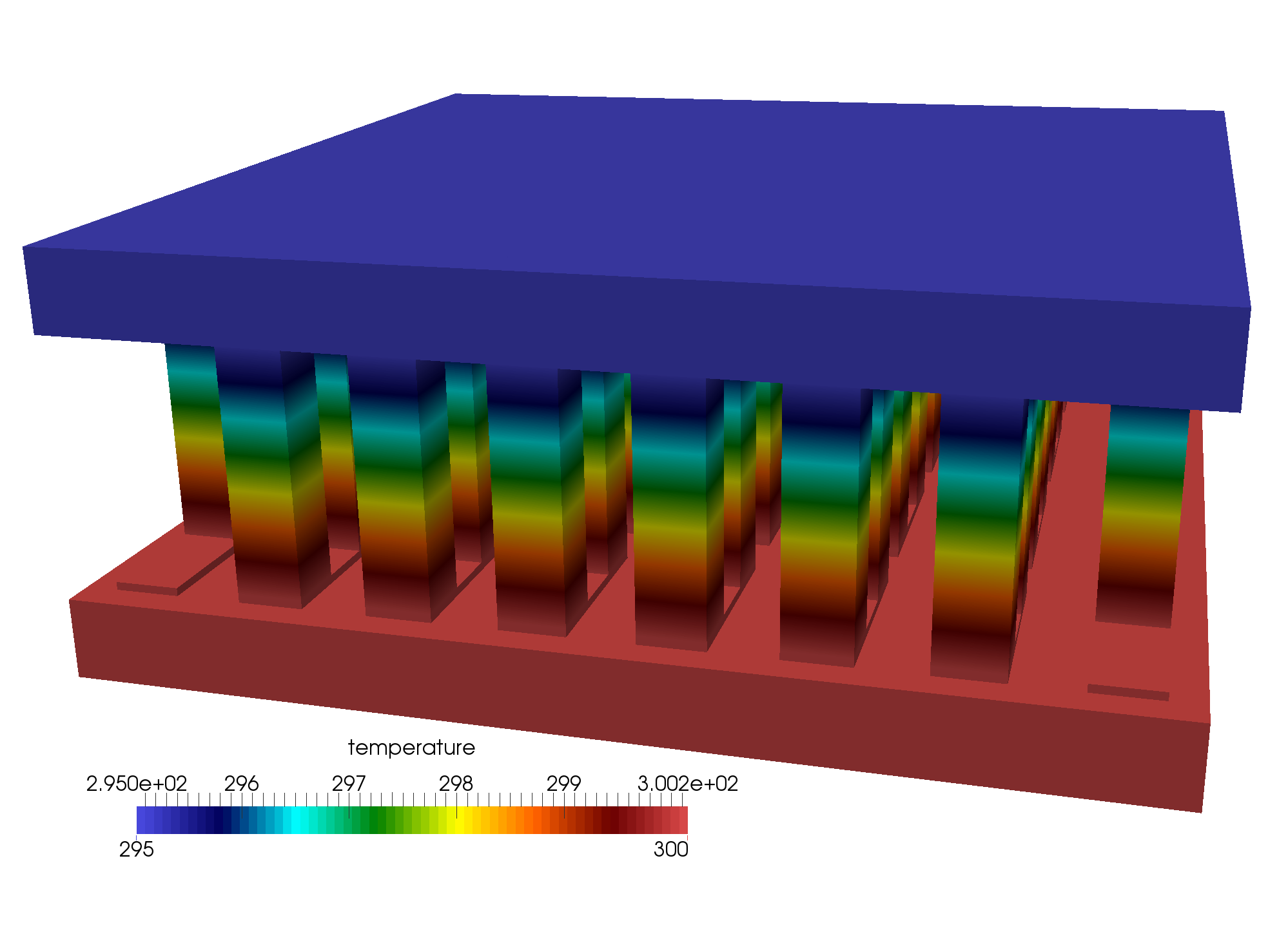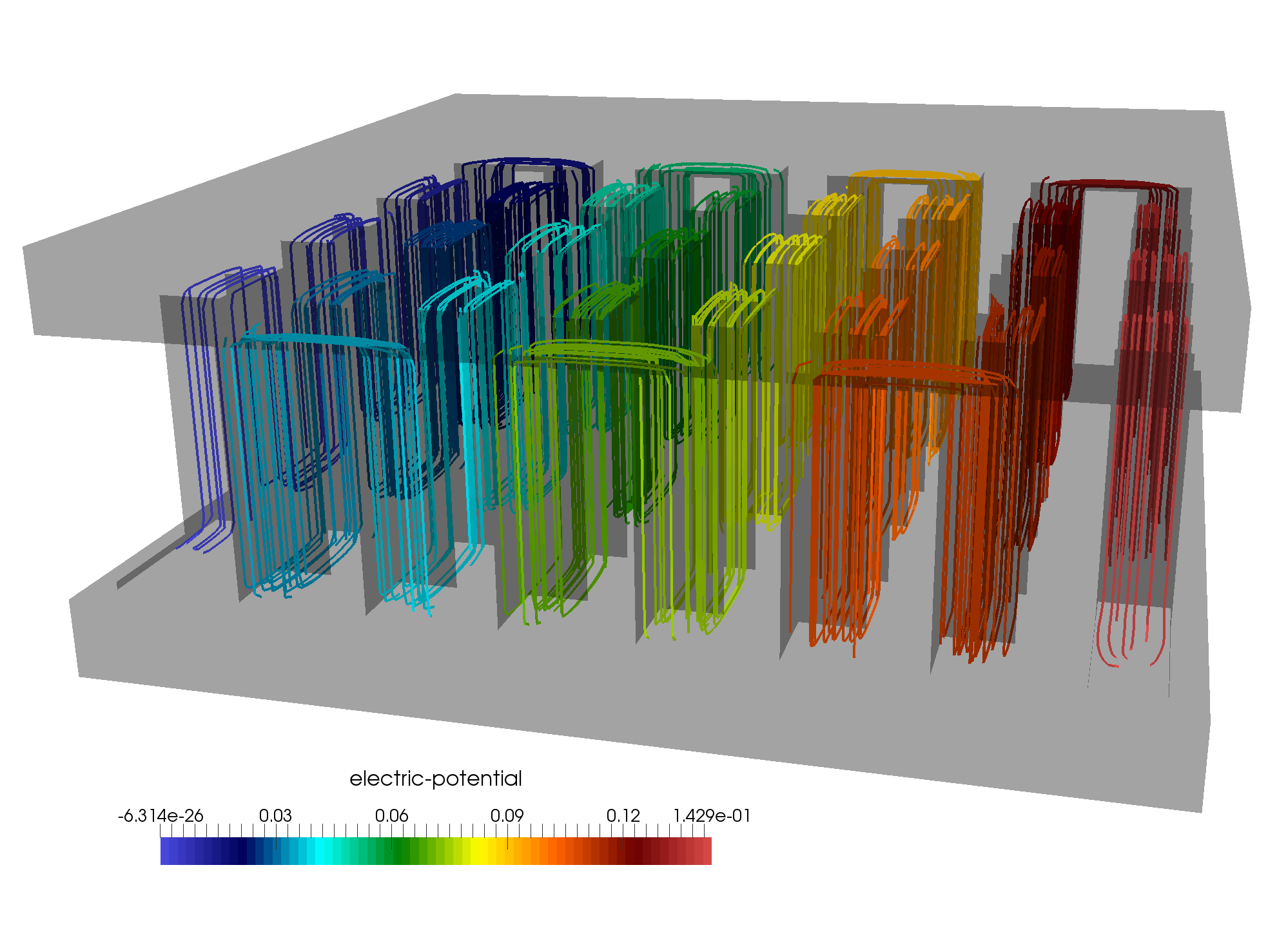# Thermoelectric Effects: Peltier Cooling and Thermoelectric Generation

## 1. Introduction

This document describes the mathematical model for the simulation of thermoelectric effects, specifically Peltier cooling and thermoelectric generation. The coupled heat equation and Poisson’s equation are extended to include the thermoelectric effects.

These effects are governed by the following equations:

\begin{align*} -\vec{\nabla}\left(\left(\sigma \alpha^2 T+\lambda\right) \vec{\nabla} T\right)-\vec{\nabla}(\sigma \alpha T \vec{\nabla} V) &= \sigma\left((\vec{\nabla} V)^2+\alpha \vec{\nabla} T \vec{\nabla} V\right) \\ \vec{\nabla}(\sigma \alpha \vec{\nabla} T)+\vec{\nabla}(\sigma \vec{\nabla} V) &= 0 \end{align*}

In these equations:

• $T$ is the temperature.

• $V$ is the voltage.

• $\alpha$ is the thermopower (Seebeck coefficient).

• $\sigma$ is the electrical conductivity.

• $\lambda$ is the thermal conductivity.

Usually, the properties $\alpha$, $\sigma$, and $\lambda$ may depend on temperature and may be anisotropic. However, in this model, we assume isotropic material properties at constant material parameters.

## 2. Material Properties

Numerical material properties are provided in the following table:

Symbols Thermoelectric Material Electrode (Copper)

Seebeck Coefficient

$\alpha$ (V/K)

p: 200e-6, n:-200e-6

6.5e-6

Electric Conductivity

$\sigma$ (S/m)

1.1e5

5.9e8

Thermal Conductivity

$\lambda$ (W/m/K)

1.6

350

Density

$\rho$ (kg/m^3)

7740

8920

Heat Capacity

$C$ (J/kg/K)

154.4

385

These properties will be used in the simulations to model the thermoelectric effects accurately.

## 3. Geometry

We consider a Peltier deviceFigure 1. Partitioning of the geometry

## 4. Results

We simulate the thermoelectric effects of Peltier cooling and thermoelectric generation. The following plots show the temperature and voltage distribution in the Peltier device.Figure 2. Temperature distributionFigure 3. Voltage distribution